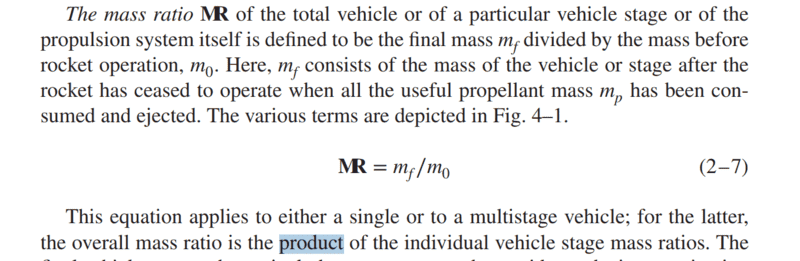# How to define the mass ratio of a multi-stage rocket?

Leo LiuSutton writes that the mass ratio of a multistage rocket is the product of the individual vehicle stage mass ratios, does it mean the expression below?
$$MR=\prod_{i} \frac{m_{f,i}}{m_{0,i}}$$

Gold Member
Yes.

Gold Member
It a bit obvious but just to make sure please note that "individual stage" here don't refer to each stage in isolation but to the rocket stack after each individual staging such that the final mass of a stage is the initial mass of next stage, i.e. ##m_{0,i+1} = m_{f,i}##.

•Leo Liu
the final mass of a stage is the initial mass of next stage...
I don't think that would be correct. The final mass of a stage contains plenty of mass which you drop before the next stage ignited. That's the very point of having multiple stages.

Gold Member
I don't think that would be correct.
For the payload ratio, the structural mass of the i-th stage is included in ##m_{0,i}##. Its both described on Multistage rocket (Wikipedia) and e.g. by Wiesel in "Spaceflight Dynamics" (using the symbols from this thread):

W.E. Wiesel said:
... the initial mass ##m_{0,k}## of the ##k##th stage is the mass of everything above the separation plane for that stage. The final mass of the ##k##th stage, ##m_{f,k}##, is the structural mass of that stage, plus the mass of stages still remaining. The ##(n+1)##th stage in an ##n## stage rocket is the payload, mass ##m_*##.

Its also easy to see that for the total payload ratio to be equal to the product of the individual payload ratios, i.e. for $$\frac{m_*}{m_{0}} \equiv \frac{m_{f,n+1}}{m_{0,1}} = \prod_{i=1}^{n+1} \frac{m_{f,i}}{m_{0,i}}$$ to hold then it must follow that ##m_{0,i+1} = m_{f,i}##.

Gold Member
My appologies.

I found my copy of Sutton's "Rocket Propulsion Elements" and can see why my statements may have caused confusion. Sutton's mass ratio (MR) is not the same as Weisel's payload ratio (##\pi##) even though both form a product and call it overall mass and payload ratio, respectively. The individual MR ratios are good for simple delta-V calculation (with ##\Delta v = -\sum_i v_{i} \ln MR_i##) while the overall payload ratio (and its associated stage structural factor) is good for optimization analysis that tries to separate "design" of each stage and the staging itself.

I can see that Sutton indeed mentions the overall mass ratio as the product shown in first post, but I do not recall any particular use for it. Anyone?

Benjies
The author has worded this definition awkwardly. I believe the mass ratio defined in the paragraph you cite is just the mass of all of the inert portions of the rocket (including payload) divided by the overall mass of the rocket on the launchpad, including propellant.

It's kind of a semantic at the end of the day, because all we care about is minimizing the mass of everything that isn't either payload or propellant (and even then, minimizing propellant mass), but assuming this question is geared toward a homework question, I believe the author is saying the mass of all inerts including payload, divided by the mass of everything on the launchpad. Double check this with your TAs- I've seen professors pull a quick one on students before.

•Leo Liu
Staff Emeritus
2022 Award
but to the rocket stack after each individual staging
Sorry, nothing to add to the thread, just wanted to say that I love the phrase "Rocket Stack". It's both very high-tech and very low-tech sounding at the same time.

•Tom.G
Mentor
I can see that Sutton indeed mentions the overall mass ratio as the product shown in first post, but I do not recall any particular use for it. Anyone?
You can plug that ratio into the (single-stage) rocket equation as effective mass ratio. The mass ratio passed by each stage combined. Between stages you have to remove the inert mass that's dropped - otherwise this would be a telescopic product and you would simply get the initial to final mass ratio, which is incorrect.

Gold Member
You can plug that ratio into the (single-stage) rocket equation as effective mass ratio.
The equivalent single-stage equation would then be $$\Delta v = -v_e \log MR,$$ with ##v_e## being the effective total ejection speed. If this has to match the multi-stage rocket equation $$\Delta v = - \sum_i v_{e,i} MR_i$$ with ##v_{e,i}## being the effective ejection speed for stage ##i##, then $$v_e = \frac{\sum_i v_{e,i}\log MR_i}{\sum_i \log MR_i}$$ which only reduce to something simple if ##v_{e,i}## is constant for all stages, i.e. if ##v_e = v_{e,i}##.

If its not (which I suspect is the general case for pretty much any launch stack due reduced ##v_{e,0}## from atmospheric pressure), then I can't quite see the benefit or analytic power of trying to express the multi-stage rocket equation as an equivalent single stage equation since you just hide the complexity in the calculation of ##v_e##. Or did I miss something?

Mentor
It only works with a constant exhaust velocity, sure. Same as the standard single-stage rocket equation, which doesn't vary the exhaust velocity either.

If you want a realistic mission profile of a launch you can't use the rocket equation anyway, you need to simulate the actual trajectory.

•Filip Larsen